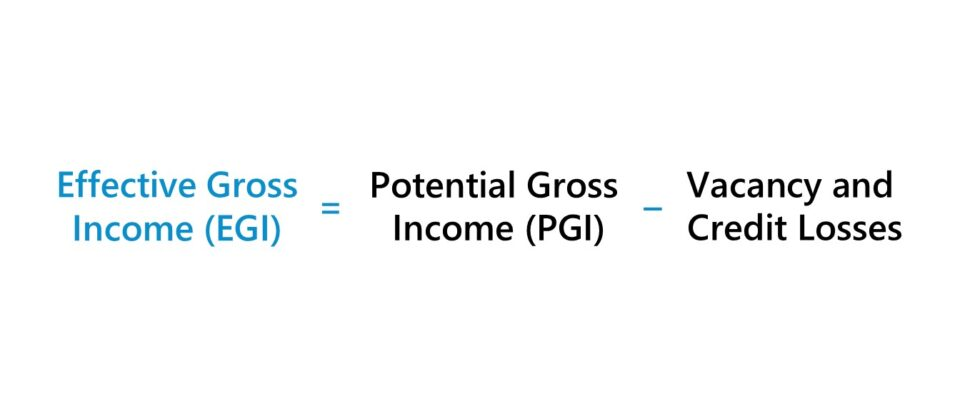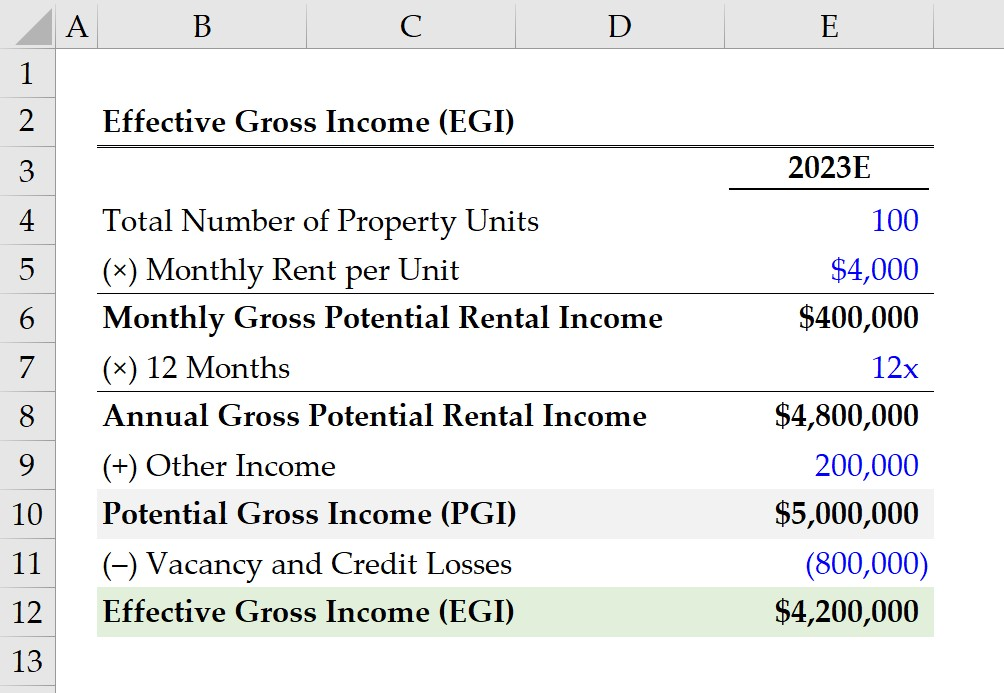Welcome to Wall Street Prep! Use code at checkout for 15% off.# Effective Gross Income (EGI)

Guide to Understanding Effective Gross Income (EGI)## How to Calculate Effective Gross Income (EGI)?

The effective gross income (EGI) is the sum of a real estate property’s total potential income less any adjustments that pertain to vacancy and credit (collection) losses.

The effective gross income (EGI) – often used interchangeably with the term “effective gross revenue” (or EGR) – measures the approximate income that a property owner can earn on a rental property investment.

The EGI of a real estate property investment offers practical insights into whether enough positive cash flows can be brought in to cover its total operating expenses and be profitable.

The composition of the property’s total potential income is, for the most part, rental income.

• Rental Income → The rental income earned on an investment property, whereby the owner leases out the property in exchange for periodic rental payments from the tenant(s) for an agreed-upon time period, is the primary source of returns.
• Other Income → However, other sources of income generated by the property, such as application fees and payments collected from tenants for usage of amenities, late fees, pet fees, and on-premise services (e.g. vending machines, laundry, dry cleaning, extra storage units, parking permits) must also be included.

Once the gross potential income is determined, the next step is to deduct any incurred costs, such as vacancy costs and credit costs.

• Vacancy Costs → The vacancy costs are the projected losses that a property owner will incur because of vacant units. The vacancy rate assumption determines the estimated vacancy costs, which is the percentage of properties (or property units) not occupied by a tenant.
• Credit Costs → The credit costs, or “collection costs”, are the losses that stem from tenants not fulfilling their obligations to pay rent. Occasionally, a property owner might not be able to collect rental income from a tenant on time, but retrieve the unmet amount (and the late fee) on a later date. If not, the tenant might not have the financial capacity to meet the payment, resulting in a default on the lease and property foreclosure.## Effective Gross Income Formula (EGI)

The formula to calculate the effective gross income (EGI) is as follows:

Effective Gross Income (EGI) = Potential Gross Income (PGI) Vacancy and Credit Losses

Where:

## Effective Gross Income (EGI) vs. Net Operating Income (NOI)

Understanding the effective gross income (EGI) is necessary to estimate a real estate property’s revenue-generating potential, namely because the EGI is part of the net operating income (NOI) calculation.

The net operating income (NOI) – widely perceived as the most important metric in the real estate industry – is relied on by investors to facilitate informed investment decisions.

Net Operating Income (NOI) = Effective Gross Income (EGI) Total Operating Expenses

The income component of the NOI formula is the effective gross income (EGI), rather than the potential gross income, i.e. post-adjustment for vacancy and credit losses.

## Effective Gross Income Calculator (EGI)

We’ll now move on to a modeling exercise, which you can access by filling out the form below.#### Excel TemplateSubmitting...

## 1. Real Estate Rental Property Assumptions

Suppose a real estate investor is attempting to estimate the effective gross income (EGI) of an existing property investment.

The property has a total of 100 units and the monthly rent per unit is \$4k.

• Total Number of Property Units = 100 Units
• Monthly Rent per Unit = \$4,000

Therefore, the monthly gross potential rental income is \$400k, which we determined by multiplying the total number of units by the monthly rent per unit. But the figure must be converted into an annual figure by multiplying it by 12 to arrive at \$4.8 million for the annual gross potential rental income.

• Monthly Gross Potential Rental Income = 100 × \$4,000 = \$400,000
• Annual Gross Potential Rental Income = \$400,000 × 12 = \$4,800,000

The next component of the potential gross income (PGI) is the other sources of income, which we’ll assume is \$200k per year.

• Other Income = \$200,000

The sum of the annual gross potential rental income and other income amounts to \$5 million, which is the total income that could be generated by the property if there were no vacancy or credit losses, i.e. if the property were at a 100% occupancy rate and has no collection issues.

• Potential Gross Income (PGI) = \$4,800,000 + \$200,000 = \$5,000,000

## 2. Effective Gross Income Calculation Example (EGI)

In the next section, we’ll adjust our potential gross income (PGI) by the estimated costs attributable to vacancy and credit losses.

The total vacancy and credit costs are forecasted to be \$800k, which the property owner determined based on historical data and performing market research, i.e. current state of the real estate market conditions (supply/demand).

• Vacancy and Credit Losses = \$800,000

In closing, our final step is to deduct the vacancy and credit losses from the potential gross income (PGI) of the property to arrive at a pro forma effective gross income (EGI) of \$4.2 million.

• Effective Gross Income (EGI) = \$5,000,000 – \$800,000 = \$4,200,00020+ Hours of Online Video Training

### Master Real Estate Financial Modeling

This program breaks down everything you need to build and interpret real estate finance models. Used at the world's leading real estate private equity firms and academic institutions.

Inline Feedbacks